# Applied Econometrics

### Course description

This course provides an introduction to basic econometric methods. These are the tools of data analysis that economists and other social scientists use to estimate the size of economic and social relationships, and to test hypotheses about them, using real-world data. The course is designed to provide the students with knowledge of theoretical econometrics and equip them with the tools and practical skills they need to critically read econometric research produced by others and to be able to conduct their own independent research.

### Course outcomes

Upon the completion of this course, students will be able to:

• Understand econometric models that include simple linear regression, multiple linear regression, discrete models and simultaneous equation models
• Understand time series econometrics and panel data regression
• Evaluate several important techniques in econometrics and apply them to economic problems
• Use statistical software to analyze data, understand and interpret results
• Evaluate the appropriateness and limitation of the methodology used in various researches

### Course contents

Click the down arrow icon [ 🔽 ] to expand and collapse the course topics.

🔽 1 h 18 min | Simple Linear Regression
• Definitions of econometrics
• Mathematical models and econometric models
• Classical assumptions of linear regression
• Ordinary Least Square (OLS) methods
• Properties of the OLS estimators
🔽 1 h 24 min | Multiple Linear Regression
• Multiple regression model
• The least squares estimates of the coefficients
• Gauss-Markov theorem
• Variance and covariance estimates and standard errors
🔽 1 h 03 min | Discrete Models
• Maximum likelihood estimation
• Regression models for binomial, multinomial and ordinal information
• Coefficients of the discrete models
🔽 1 h 18 min | Simultaneous Equation Models
• Simultaneous equation modeling
• The problem of endogeneity (simultaneity and its causes)
• Concept of identifiability of equations in simultaneous equations modeling
• Alternative estimation methods of simultaneous equations
🔽 1 h 47 min | Time Series Econometrics
• Lags in time-series models
• Multiple regression assumptions modified to accommodate time series data
• Data generating process of time series
• Engle-Granger and Johansen co-integration tests using real data
• Concept of co-integration and error correction mechanism
🔽 0 h 42 min | Panel Data Regression
• Basic concepts of panel data econometrics
• Fixed effect models
• Random effect models

### This course includes: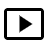7 h 32 min recorded video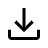Downloadable resources (books and articles)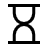One year access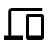Access on mobile and TV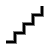Advanced Level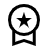Certificate of completion

#### Self-paced

7,900 Br
1 year of access
This course does not have any sections.